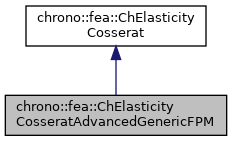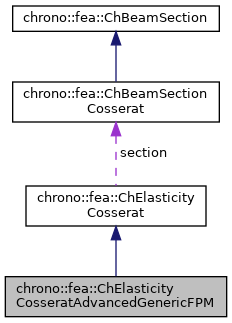## Description

A Cosserat section which allows to input the material fully-populated stiffness matrix(FPM) of cross-section directly.

#include <ChBeamSectionCosserat.h>[legend][legend]

## Public Member Functions

ChElasticityCosseratAdvancedGenericFPM (const ChMatrixNM< double, 6, 6 > mKlaw, const double malpha, const double mCy, const double mCz, const double mbeta, const double mSy, const double mSz)

virtual void SetEMatrix (const ChMatrixNM< double, 6, 6 > &mKlaw)
Set the FPM section & material of beam element, giving the stiffness FPM directly.

virtual ChMatrixNM< double, 6, 6 > & GetEMatrix ()
Get the stiffness FPM of section & material of beam element.

void SetSectionRotation (double ma)
Set the rotation in [rad] of the Y Z axes for which the YbendingRigidity and ZbendingRigidity values are defined.

double GetSectionRotation ()
Get the rotation in [rad] of the Y Z axes.

void SetCentroid (double my, double mz)
"Elastic reference": set the displacement of the elastic center (or tension center) respect to the reference section coordinate system placed at centerline.

double GetCentroidY ()

double GetCentroidZ ()

void SetShearRotation (double mb)
Set the rotation in [rad] of the Y Z axes for which the YshearRigidity and ZshearRigidity values are defined.

double GetShearRotation ()

void SetShearCenter (double my, double mz)
"Shear reference": set the displacement of the shear center S respect to the reference beam line placed at centerline. More...

double GetShearCenterY ()

double GetShearCenterZ ()

void UpdateEMatrix ()
Need to update the material stiffness matrix Klaw after input section rotation and elastic center/shear center offset. More...

virtual ChMatrixNM< double, 6, 6 > & GetTransformMatrix ()
Get the tranformation matrix of seciton, may be useful for debug.

virtual void ComputeStress (ChVector<> &stress_n, ChVector<> &stress_m, const ChVector<> &strain_e, const ChVector<> &strain_k) override
Compute the generalized cut force and cut torque. More...

virtual void ComputeStiffnessMatrix (ChMatrixNM< double, 6, 6 > &K, const ChVector<> &strain_e, const ChVector<> &strain_k) override
Compute the 6x6 tangent material stiffness matrix [Km] = dσ/dε. More...Public Attributes inherited from chrono::fea::ChElasticityCosserat
ChBeamSectionCosseratsection

## Constructor & Destructor Documentation

 chrono::fea::ChElasticityCosseratAdvancedGenericFPM::ChElasticityCosseratAdvancedGenericFPM ( const ChMatrixNM< double, 6, 6 > mKlaw, const double malpha, const double mCy, const double mCz, const double mbeta, const double mSy, const double mSz )
inline
Parameters
 mKlaw 6x6 material fully-populated stiffness matrix(FPM) of cross-section malpha rotation of reference at elastic center, for bending effects [rad] mCy elastic center y displacement respect to centerline mCz elastic center z displacement respect to centerline mbeta rotation of reference at shear center, for shear effects [rad] mSy shear center y displacement respect to centerline mSz shear center z displacement respect to centerline

## ◆ ComputeStiffnessMatrix()

 void chrono::fea::ChElasticityCosseratAdvancedGenericFPM::ComputeStiffnessMatrix ( ChMatrixNM< double, 6, 6 > & K, const ChVector<> & strain_e, const ChVector<> & strain_k )
overridevirtual

Compute the 6x6 tangent material stiffness matrix [Km] = dσ/dε.

Parameters
 K 6x6 stiffness matrix strain_e local strain (deformation part): x= elongation, y and z are shear strain_k local strain (curvature part), x= torsion, y and z are line curvatures

Reimplemented from chrono::fea::ChElasticityCosserat.

## ◆ ComputeStress()

 void chrono::fea::ChElasticityCosseratAdvancedGenericFPM::ComputeStress ( ChVector<> & stress_n, ChVector<> & stress_m, const ChVector<> & strain_e, const ChVector<> & strain_k )
overridevirtual

Compute the generalized cut force and cut torque.

Parameters
 stress_n local stress (generalized force), x component = traction along beam stress_m local stress (generalized torque), x component = torsion torque along beam strain_e local strain (deformation part): x= elongation, y and z are shear strain_k local strain (curvature part), x= torsion, y and z are line curvatures

Implements chrono::fea::ChElasticityCosserat.

## ◆ SetShearCenter()

 void chrono::fea::ChElasticityCosseratAdvancedGenericFPM::SetShearCenter ( double my, double mz )
inline

"Shear reference": set the displacement of the shear center S respect to the reference beam line placed at centerline.

For shapes like rectangles, rotated rectangles, etc., it corresponds to the elastic center C, but for "L" shaped or "U" shaped beams this is not always true, and the shear center accounts for torsion effects when a shear force is applied.